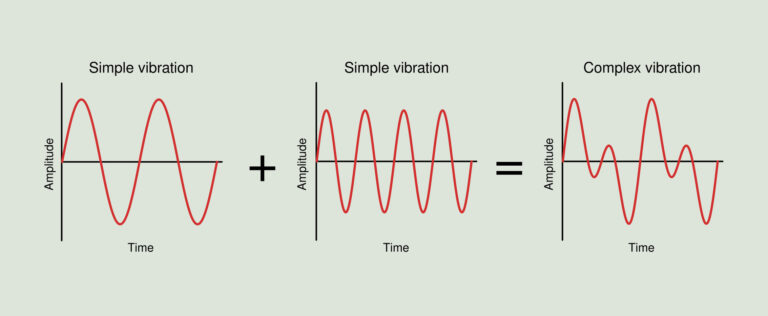vibration notes pdf| vibration notes pdf | vibration notes in pdf | Handwritten notes Mechanical Engineering | Written by Ashish SirVibration is a mechanical phenomenon whereby oscillations occur about an equilibrium point. The word comes from Latin vibrationem. The oscillations may be periodic, such as the motion of a pendulum—or random, such as the movement of a tire on a gravel road

Vibration notes Syllabus

1.Introduction : Periodical motion, harmonic motion, the vector method of representing vibrations, displacement, velocity and acceleration in harmonic motion, work done in harmonic motion, superposition of simple harmonic motion, beat phenomenon, non harmonic periodic motions. Harmonic analysis System having single degree of freedom, free vibration of systems without damping, Equilibrium and Energy Method for determining natural frequency. Reyleigh’s Method, Equivalent Systems (systems with compound springs, shafts of different diameter Equivalent length, effects of mass of spring and shaft). Free vibration of systems with Viscous, Coulomb and Structural damping. Equations of motion – Discussion of its solutions. Electrical Analogies : Electric circuit principles, equivalent circuits.

2. Forced vibrations of systems with and without damping (viscous and coulomb), Method of complex algebra, equivalent viscous damping, impressed force due to unbalance, inadmissibility, support motion, Vibration isolation, commercial isolators.

3. System with two-degree of freedom : Normal mode vibrations, Torsional systems, Coupled vibrations, General solution in terms of normal mode, vehicle suspension, Undamped dynamic vibration absorber, Centrifugal absorber, friction damper. Whirling of shafts : Whirling of light flexible shaft with an unbalance disk at the centre of its length with and without damping, discussion of the speeds above and below the critical speed, uniform shaft with and without unbalanced masses attached along its length (by Rayleigh Method) for simply supported and fixed ends.

4. Multiple degree of freedom system, introduction, modelling of continuous system as multiple degree of freedom system, newton’s law to derive equation of motion, influence coefficients, equation of motion of undamped system in matrix form, Eigen value problems and solutions., Free vibration of undamped system, Forced vibration of undamped system and viscous damped system.

5. Vibration Measurement : Principle of frequency, amplitude, velocity and acceleration measuring instruments, frequency response plots, phase shift plots, analysis of vibration records.

ChAPTER-1

1.Introduction : Periodical motion, harmonic motion, the vector method of representing vibrations, displacement, velocity and acceleration in harmonic motion, work done in harmonic motion, superposition of simple harmonic motion, beat phenomenon, non harmonic periodic motions. Harmonic analysis System having single degree of freedom, free vibration of systems without damping, Equilibrium and Energy Method for determining natural frequency. Reyleigh’s Method, Equivalent Systems (systems with compound springs, shafts of different diameter Equivalent length, effects of mass of spring and shaft). Free vibration of systems with Viscous, Coulomb and Structural damping. Equations of motion – Discussion of its solutions. Electrical Analogies : Electric circuit principles, equivalent circuits.